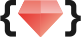# 五倍紅寶石 ・專業程式教育機構### 目錄

• Scope 介紹
• 什麼是 Scope ?
• Scope Gate
• Block 介紹
• Block 從頭開始
• Proc 基本介紹
• procs 和 lambdas 怎麼分？！
• & 符號解析
• Block 實作
• 自己做一個 before action
• 第一步：試試看在 before 中執行 block
• 第二步：在執行 before 的時候只有紀錄…
• 第三步：重新定義要執行 before 的 method
• 第四部：只有第一次執行 execute_before
• 結論

### Scope 介紹

#### 什麼是 Scope ?

``````x = 10
def method
puts x
end

method
``````

``````test.rb:3:in 'method': undefined local variable or method 'x' for main:Object (NameError)
from test.rb:6:in '<main>'
``````

``````v1 = 1
class MyClass
v2 = 2
local_variables #[:v2]
def my_method
v3 = 3
local_variables #[:v3]
end
local_variables # [:v2]
end
local_variables # [:v1, :obj]
obj = MyClass.new
obj.my_method
``````

local_variables 會回傳現在的 scope 中可以看到的區域變數

#### Scope Gates

``````my_var = "Success"
MyClass = Class.new do
puts "#{my_var} in the class definition"
define_method :my_method do
puts "#{my_var} in the method"
end
end

MyClass.new.my_method``````

``````Success in the class definition
Success in the method``````

### Block 介紹

#### Block 從頭開始

``````def my_method
x = "Goodbye"
yield("cruel")
end

x = "Hello"

my_method do |y|
puts "#{x}, #{y} world"
end ``````

``Hello, cruel world``

bindings 指的是當下 scope 可以使用的 variable, methos 還有 self

#### Proc 基本介紹

``````inc = Proc.new {|x| x + 1 }
inc.call(2) # 回傳 3
``````

#### procs 和 lambdas 怎麼分？！

``````l1 = lambda {|x| x + 1 }
l2 = ->(x){ x + 1 }
p1 = Proc.new {|x| x + 1 }
p2 = proc {|x| x + 1 }

puts "l1: #{l1.lambda?}, #{l1.class}" # l1: true, Proc
puts "l2: #{l2.lambda?}, #{l2.class}" # l2: true, Proc
puts "p1: #{p1.lambda?}, #{p1.class}" # p1: false, Proc
puts "p2: #{p2.lambda?}, #{p2.class}" # p2: false, Proc
``````

##### procs 和 lambdas 有不同的 return
``````def double(callable_object)
callable_object.call * 2
end

# return from lambdas
la = lambda { return 10 }

# return from the scope which defined the procs(main)
pr = proc { return 10 }

puts double(la) # 20
puts double(pr) # LocalJumpError...``````

`````` 20
test.rb:12:in `block in <main>': unexpected return (LocalJumpError)
from p92.rb:22:in `double'
from p92.rb:29:in `<main>'``````

lambda 的 return 是從 lambda return，而 proc 則是從定義 proc 的 scope return 意思就是如果把 proc 用一個方法包起來的話...

``````def lambda_double
la = lambda { return 10 }
result = la.call
return result * 2
end

def proc_double
pr = Proc.new { return 10 }
result = pr.call
return result * 2  # unreachable code!
end

puts lambda_double # => 20
puts proc_double   # => 10``````

`lambda_double` 可以執行完方法中的每行程式，而 `proc_double` 則會在 `pr.call` 那行就 return 了，因為 proc 的 return 是從定義 proc 的地方也就是 `proc_double` 出發的。

##### procs 和 lambdas 處理參數的方式

procs 處理參數的方式比較有彈性，而 lambdas 則是比較嚴格，說起來不清楚!? 看 code 最快

``````puts "以下是 proc"
pr = proc {|a,b| [a,b]}
p pr.call('a', 'b')
p pr.call('a')
p pr.call('a', 'b', 'c')

puts "以下是 lambda"
la = lambda {|a,b| [a,b]}
p la.call('a', 'b')
p la.call('a')
p la.call('a', 'b', 'c')``````

``````以下是 proc
["a", "b"]
["a", nil]
["a", "b"]

["a", "b"]
test.rb:8:in `block in <main>': wrong number of arguments (given 1, expected 2) (ArgumentError)
from test.rb:10:in `<main>'``````

procs 遇到多的參數會自動丟掉，而不夠就補 nil，lambdas 則要求參數的數量正確才能執行。

#### & 符號解析

& 符號可以把 block 轉成 Proc 或是轉回來，多說無益看 code 就知道

``````def second_method
puts 'In second_method'
yield
end

def first_method(&operator) # 這裡的 & 是把 block 轉成 Proc
puts 'In first_method'
operator.call
second_method(&operator) # 這裡的 & 是把 Proc 轉成 block
end

first_method do
puts "this is block"
end``````

``````In first_method
this is block
In second_method
this is block``````

### Block 實作:

#### 自己做一個 before action

``````class Example
extend BeforeAction
before :method_a, :method_b do
puts 'the code before method'
end

def method_a
puts 'this is method a'
end

def method_b
puts 'this is method b'
end
end

instance_1 = Example.new
instance_1.method_a
instance_2 = Example.new
instance_2.method_b``````

``````the code before method
this is method a
the code before method
this is method b
``````

#### 第一步：試試看在 before 中執行 block

``````module BeforeAction
def before(*methods, &block)
methods.each do |method|
block.call
end
end
end``````

#### 第二步：在執行 before 的時候只有紀錄...

``````module BeforeAction
def new
execute_before
super
end

def before(*methods, &block)
# 先存起來之後用
@methods = methods
@block = block
end

def execute_before
# to do ...
end
end``````

#### 第三步：重新定義要執行 before 的 method

``````module BeforeAction
...
def execute_before
@methods.each do |method|
# 幫原本的 method 取一個新名字
newname = "new_#{method}"
alias_method newname, method
block = @block
# 重新定義每個 method 要做什麼
define_method method do
# 執行 block
block.call
# 原本的 method
send(newname)
end
end
end
end``````

``````instance_1 = Example.new
instance_1.method_a
instance_1.method_b``````

``````the code before method
this is method a
the code before method
this is method b
``````

#### 第四部：只有第一次執行 execute_before

``````module BeforeAction
def new
execute_before if first_time?
super
end

def first_time?
return false if @not_first_time
@not_first_time = true
end

...
end

``````

#### 最後：大功告成

``````module BeforeAction
def new
# 只有第一次執行 new 時要 execute_before
execute_before if first_time?
super
end

def first_time?
return false if @not_first_time
@not_first_time = true
end

def before(*methods, &block)
# 先存起來之後用
@methods = methods
@block = block
end

def execute_before
@methods.each do |method|
# 幫原本的 method 取一個新名字
newname = "new_#{method}"
alias_method newname, method
block = @block
# 重新定義每個 method 要做什麼
define_method method do
# 執行 block
block.call
# 原本的 method
send(newname)
end
end
end
end

class Example
extend BeforeAction
before :method_a, :method_b do
puts 'the code before method'
end

def method_a
puts 'this is method a'
end

def method_b
puts 'this is method b'
end
end

instance_1 = Example.new
instance_1.method_a
instance_2 = Example.new
instance_2.method_b``````

``````the code before method
this is method a
the code before method
this is method b``````

### 結論

👩‍🏫 課務小幫手：

✨ 想掌握 Ruby on Rails 觀念和原理嗎？